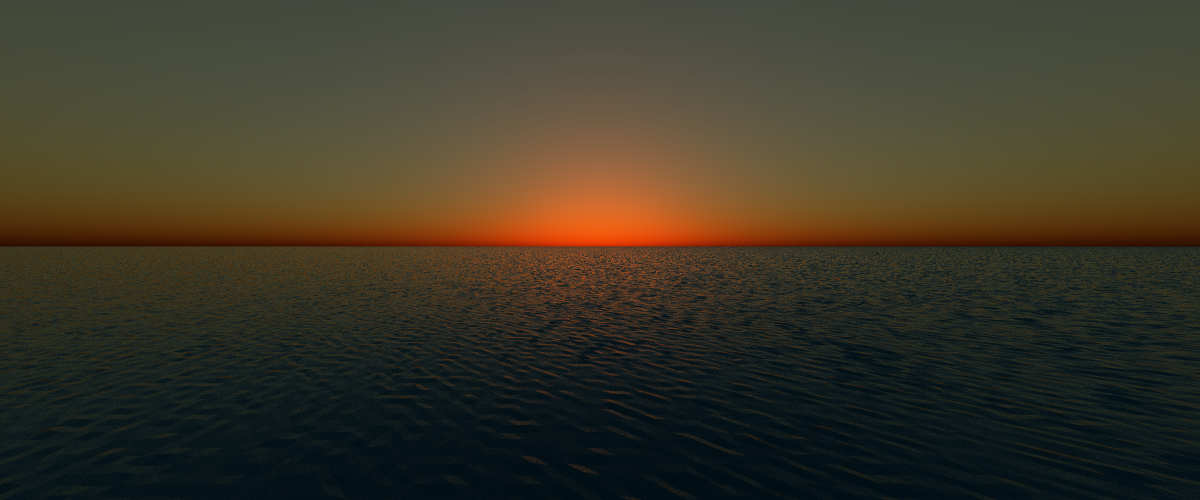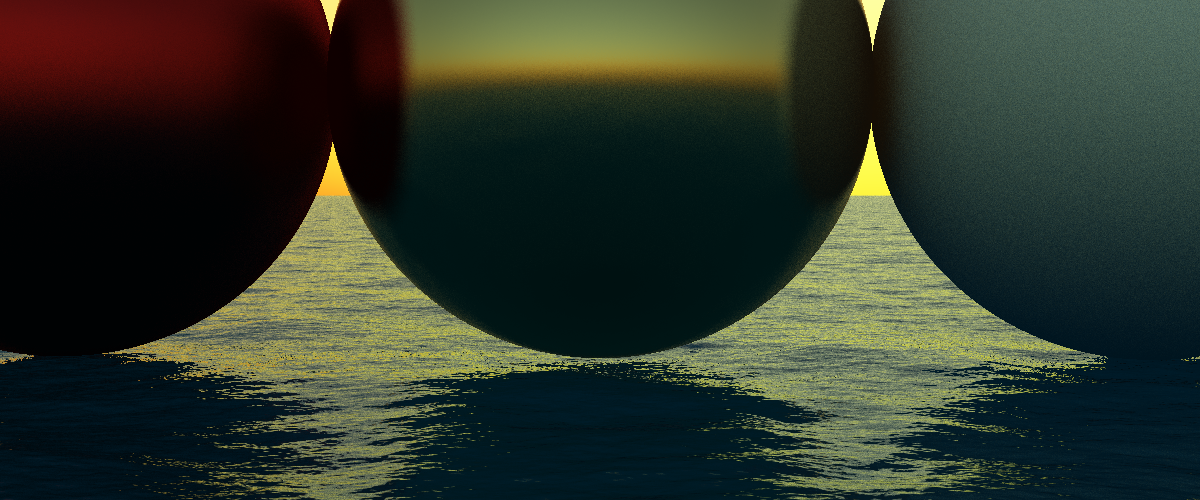//# Simulating Ocean Waves

Recently I wanted to add ocean water to my raytracer, and after some reading, found that the state of the art in Ocean water simulation is a paper called "Simulating Ocean Water" by Jerry Tessendorf.

I spent many evenings working through the paper trying to come up with an implementation.

The concept for Tessendorf's algorithm is conceptually very simple, although it takes a lot of context to get to the point where the simplicity is obvious. In this post, I hope to elucidate some of that context, for the next person to try and implement this algorithm.

I also include some of my source code. All the source I found online had been written for GPU shaders, and I wanted to create a CPU implementation, so this code was written from scratch.

The first part of the puzzle is to think about the process of wave formation. In the deep ocean, waves are formed by the flow of wind over the surface of the water. These waves travel huge distances, but the surface of the water is affected not just by the current wind, but the thousands of gusts and winds that came before. There isn't a single wave, but a large number of slightly different ones travelling through the same water.

We can describe this set of waves as a spectrum. Just like the colour of the light that you see depends on the sum of the frequencies in that light, the set of sub-waves in a patch of ocean adds up to the visible waves.

Over the years oceanographers have studied these spectra, and come up with formulae to model them. One of these is Phillips spectrum, which generates an amplitude for a wave based on a vector, a wind vector and gravity.

/// Phillips Spectrum
fn phillips(
k: Vector2<f64>,
wind: Vector2<f64>,
scale: f64,
gravity: f64
) -> f64 {

let ksq = k.x * k.x + k.y * k.y;
if ksq < std::f64::MIN_POSITIVE { return 0. };
let wind_dir = wind.normalize();
let wk = k.normalize().dot(&wind_dir);
let wind_speed = wind.norm();
let l = (wind_speed * wind_speed) / gravity;
return scale * (-1.0 / (ksq * l * l)).exp() / (ksq * ksq) * wk * wk ;
}


Of course nature isn't regular, so Tessendorf suggests we add some noise to this amplitude.

fn amplitude(
k: Vector2<f64>,
wind: Vector2<f64>,
scale: f64,
gravity: f64
) -> Complex<f64> {

return
1f64/(2f64.sqrt()) *
Complex::new(randn(), randn()) *
phillips(k, wind, scale, gravity).sqrt()
;
}


We can generate a tile of Phillips weights by creating a vector for each point on the grid. This vector represents the wave that travels from the origin (the center of the grid) to that point in a unit of time.

One of the things that I found puzzling in the paper was how we go from discussing sine-waves, to then all of a sudden introducing a formula including complex numbers. Perhaps this is obvious to mathematicians, but the missing piece of context that I lacked here was Euler's formula.

Euler was a Swiss mathematician in the 18th century who discovered that there is a link between the natural exponent and the angle between complex numbers:

eiθ=cos(θ)+isin(θ)

For the angle pi, this reduces to the famous formula:

eiπ+1=0

The way this formula becomes useful is based on it's derivation - the angle, θ, that we are describing here is the angle on a circle on the real-imaginary number plane. As the angle rotates between the real and imaginary, so the real and imaginary components of the exponential describe the location of the point.

This is very useful to us, because we can therefore describe a circular wave in time with only a single complex number.

It also leads to Tessendorf's key insight - because we can describe the spectrum of waves as a field of complex numbers, we can sum these waves with an inverse Fourier transform. And as we have a discrete set of points on a mesh that we are generating for a tile of the ocean surface, the problem is actually a Discrete Fourier Transform, which has an efficient implementation - the Fast-Fourier Transform.

This means that we can generate the heights of the ocean surface on a tile with size n in O(nlogn) time, rather than O(n2).

The nice thing about this mesh is that it tiles perfectly because the waves are naturally repeating over the amplitude of the tile-size. We can therefore repeat the mesh to form an infinite plane of ocean texture, although the repetitiveness will look unnatural, therefore we must pick our viewpoint carefully.

Full code is on github